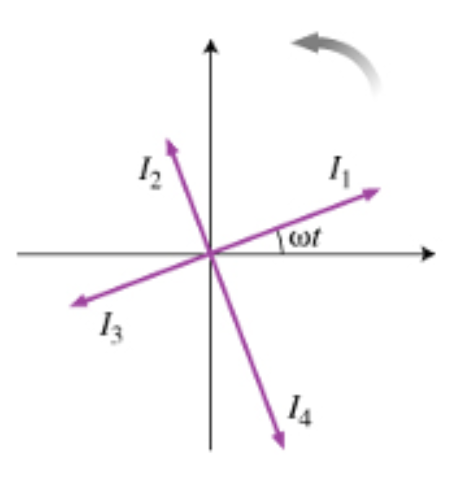# Problem: Let us now consider some basic applications of phasors to AC circuits. For a resistor, the current and the voltage are always in phase. For an inductor, the current lags the voltage by π/2For a capacitor, the current leads the voltage by π/2Consider this diagram. Let us assume that it describes a series circuit containing a resistor, capacitor, and an inductor. The current in the circuit has amplitude, I, as indicated in the figure.Which of the following choices gives the correct respective labels of the voltage across the resistor, the capacitor and the inductor? A) V1; V2; V3B) V1; V2; V4C) V1; V4; V2D) V3; V2; V4E) V3; V4; V2

###### FREE Expert Solution

VL and VC are out of phase by π.

4 is lagging 2, thus C and E are incorrect.

79% (121 ratings)###### Problem Details

Let us now consider some basic applications of phasors to AC circuits.

• For a resistor, the current and the voltage are always in phase.
• For an inductor, the current lags the voltage by π/2
• For a capacitor, the current leads the voltage by π/2Consider this diagram. Let us assume that it describes a series circuit containing a resistor, capacitor, and an inductor. The current in the circuit has amplitude, I, as indicated in the figure.

Which of the following choices gives the correct respective labels of the voltage across the resistor, the capacitor and the inductor?

A) V1; V2; V3

B) V1; V2; V4

C) V1; V4; V2

D) V3; V2; V4

E) V3; V4; V2